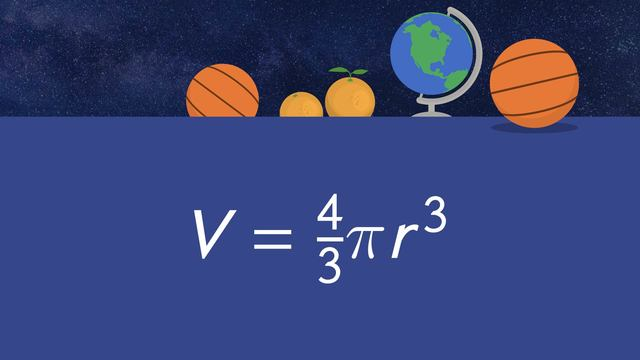# Volume of a SphereRating

Be the first to give a rating!
The authorsTeam Digital

## Basics on the topicVolume of a Sphere

Volume of a Sphere - Using the formula, and given a radius, you can find the volume of a sphere.

## Volume of a Sphere exercise

Would you like to apply the knowledge you’ve learned? You can review and practice it with the tasks for the video Volume of a Sphere.
• ### Determine which formula would be used to find the volume of the sphere.

Hints

The radius of a circular object is the measurement from the center to the outer edge, while the diameter is the measurement from one side to the other, passing through the center.

To find the volume, the radius needs to be substituted into the formula for $r$. Substitution means you are replacing a variable with a known value.

Solution

The formula used to find the volume of the volleyball would be:

$V=\frac{4}{3}\pi (4^3)$

• ### Determine the sequence of events to solve for the volume of a sphere.

Hints

The first step when finding the volume of any shape is to identify the formula you will use.

After identifying the formula, find the radius, $r$, and substitute it into $V=\frac{4}{3} \pi r^3$.

Don't forget... in terms of pi means you leave $\pi$ as a symbol rather than calculating it.

Solution

Step 1: Identify the formula and radius.

$V = \frac{4}{3}\pi r^3$

$r=9$

Step 2: Substitute the radius in for $r$ in the formula.

$V = \frac{4}{3}\pi (9^3)$

Step 3: Evaluate the exponent.

$V = \frac{4}{3}\pi (729)$

Step 4: Multiply the known values, other than $\pi$.

$V = 972\pi$

Step 5: Leave $\pi$ as a symbol since the directions state to leave in terms of pi, and add the appropriate units.

$V = 972\pi\:cm^3$

• ### Show your understanding of the relationship between a radius and a diameter of a sphere.

Hints

The radius and diameter are labeled on the sphere.

The radius of a sphere is half the distance of the diameter.

Solution

The diameter is the distance across a sphere through its center and the radius is the distance from the center to the outside. The radius is exactly half the distance of a diameter. The formula to find the volume of a sphere is $V=\frac{4}{3}\pi r^3$, which means the radius is the information needed. Given the diameter, we can find the radius, by $\bf{\dfrac{\text{diameter}}{2}}$. The sphere has a diameter of 16 inches and a radius of 8 inches.

• ### Find the volume of a sphere.

Hints

After you have substituted the value of the radius in the $r$, cube it, and multiply that by $\frac{4}{3}$.

This solution should not be rounded.

The product of $r^3$ and $\frac{4}{3}$ can then be multiplied by $\pi$ using a calculator.

Solution

$V=\frac{4}{3}\pi (4^3)$

$V=\frac{4}{3}\pi (64)$

$\frac{4}{3}(64) = 85 \frac{1}{3}$

$V=85\frac{1}{3}\pi$

$V=268.082573...$

$V\approx268.1\:cm^3$

• ### Identify the formula used to find the volume of a sphere.

Hints

The Volume of a Sphere refers to the space inside the sphere.

The radius of a sphere refers to the distance from the center of the sphere to any point on its surface.

It is always the same length regardless of the direction in which it is measured.

If the radius of the sphere was 5cm, we could substitute it for the $r$ in the formula for volume, like this...

$V=\frac{4}{3} \pi (5^3)$

Solution

The volume of a sphere can be calculated using this formula.

$V=\frac{4}{3}\pi r^3$

• ### Demonstrate your knowledge on finding the volume of a sphere.

Hints

The volume of a sphere formula is:

$V = \frac{4}{3}\pi r^3$

The radius is half of the diameter.

Be sure to check each choice to see if the diameter or radius will yield a sphere with a volume of $1,767.1\:cm^3$.

Solution

$\text{diameter}=15\:cm$
$\text{radius}=7.5\:cm$